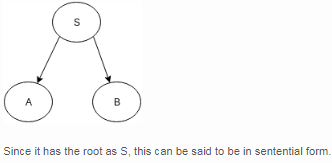Courses

# Test: DPDA And Context Free Languages

## 10 Questions MCQ Test Theory of Computation | Test: DPDA And Context Free Languages

Description
This mock test of Test: DPDA And Context Free Languages for Computer Science Engineering (CSE) helps you for every Computer Science Engineering (CSE) entrance exam. This contains 10 Multiple Choice Questions for Computer Science Engineering (CSE) Test: DPDA And Context Free Languages (mcq) to study with solutions a complete question bank. The solved questions answers in this Test: DPDA And Context Free Languages quiz give you a good mix of easy questions and tough questions. Computer Science Engineering (CSE) students definitely take this Test: DPDA And Context Free Languages exercise for a better result in the exam. You can find other Test: DPDA And Context Free Languages extra questions, long questions & short questions for Computer Science Engineering (CSE) on EduRev as well by searching above.
QUESTION: 1

### Context free grammar is called Type 2 grammar because of ______________ hierarchy.

Solution:

Chomsky hierarchy decide four type of language :Type 3- Regular Language, Type 2-Context free language, Type 1-Context Sensitive Language, Type 0- Unrestricted or Recursively Ennumerable language.

QUESTION: 2

### a→bRestriction: Length of b must be atleast as much length of a.Which of the following is correct for the given assertion?

Solution:

A context-sensitive grammar (CSG) is a formal grammar in which the left-hand sides and right-hand sides of any production rules may be surrounded by a context of terminal and non terminal symbols. Context-sensitive grammars are more general than context-free grammars, in the sense that there are some languages that cannot be described by context-free grammars, but can be described by CSG.

QUESTION: 3

### From the definition of context free grammars, G=(V, T, P, S) What is the solution of VÇT?

Solution:

V is the set of non terminal symbols while T is the st of terminal symbols, their intersection would always be null.

QUESTION: 4

If P is the production, for the given statement, state true or false.P: V->(V∑T)* represents that the left hand side production rule has no right or left context.

Solution:

Here the production P is from the definition of Context free grammar and thus, has no right or left context.

QUESTION: 5

There exists a Context free grammar such that:X->aXWhich among the following is correct with respect to the given assertion?

Solution:

The grammar with right recursive production is known as Right recursive grammar. Right recursive production is of the form X->aX where a is a terminal and X is a non terminal.

QUESTION: 6

If the partial derivation tree contains the root as the starting variable, the form is known as:

Solution:

Example: For any grammar, productions be:
S->AB
A->aaA| ^
B->Bb| ^
The partial derivation tree can be drawn as:QUESTION: 7

Find a regular expression for a grammar which generates a language which states :
L contains a set of strings starting wth an a and ending with a b, with something in the middle.

Solution:

The grammar for the same language can be stated as :
(1) S → aMb
(2) M → A
(3) M → B
(4) A → e
(5) A → aA
(6) B → e
(7) B → bB

QUESTION: 8

Which of the following is the correct representation of grammar for the given regular expression?
a(aUb)*b

Solution:

The basic idea of grammar formalisms is to capture the structure of string by
a) using special symbols to stand for substrings of a particular structure
b) using rules to specify how the substrings are combined to form new substrings.

QUESTION: 9

A CFG consist of the following elements:

Solution:

A CFG consists of:
a) a set of terminals, which are characters of alphabets that appear in the string generated by the grammar.
b) a set of non terminals, which are placeholders for patterns of terminal symbols that can be generated by the nonterminal symbols.
c) a set of productions, which are set of rules to transit from one state to other forming up the string
d) a start symbol, a special non terminal symbol that appears in the initial string generated in the grammar.

QUESTION: 10

A CFG for a program describing strings of letters with the word “main” somewhere in the string:

Solution:

None.